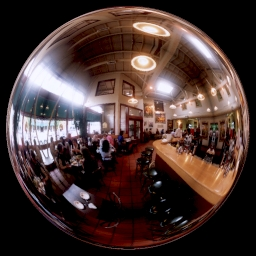# Emulate GLSL texture sphere mapping with Optix

Hi, I’m trying to convert my GLSL shaders to Optix.

My GLSL code creates Spherical Mapping texture coordinates to obtain an image like this (environmental texture mapping)I have some troubles with this conversion (see GLSL VERTEX shader code below)

My problem is to obtain an Optix equivalent of this two values (gl_NormalMatrix and gl_ModelViewMatrix)

``````vec3 NormalEye = gl_NormalMatrix * gl_Normal;
vec4 PositionVec4 = gl_ModelViewMatrix * gl_Vertex;
vec3 PositionEye = PositionVec4.xyz;
``````

Here is the full GLSL code

Thank you
RD

``````vec4 SphereMap(in vec3 normal, in vec3 ecPosition3)
{
float  m;
vec3   r,u;

u = normalize(ecPosition3);
r = reflect(u, normal);
m = 2.0 * sqrt(r.x * r.x + r.y * r.y + (r.z + 1.0) * (r.z + 1.0));

return vec4 (r.x / m + 0.5, r.y / m + 0.5, 0.0, 1.0);
}

vec3 NormalEye = gl_NormalMatrix * gl_Normal;
vec4 PositionVec4 = gl_ModelViewMatrix * gl_Vertex;
vec3 PositionEye = PositionVec4.xyz;

// Sphere Mapping
gl_TexCoord = SphereMap( NormalEye, PositionEye );
``````

From a ray tracing quality point of view I would not recommend to use that sphere map projection at all.

The sphere map is meant to work for a rasterizer which basically does primary rays only.
The texture projection on that circular fisheye-like texture image is mapping the whole backside of the surrounding to the silhouette of that image.
Means if you use that texture in a ray tracer in reflections which have direction vectors pointing to the camera, there won’t be sufficient texture information to result in a nice looking image, at least not compared to cube maps or spherical environment maps.

If you’re still intending to use them, here are the next problems:

OpenGL is working in eye-coordinates after the transformations with the modelview and normal matrices. Latter is the inverse transpose of the modelview matrix.
The modelview matrix is the concatenation of the transform matrix from object to world space and the transform matrix from world to eye-space.
Means in OpenGL the transformation is from object space directly to eye-space. The eye position is at the origin (0, 0, 0) in eye-space, which is right-handed and looking down the negative z-axis.

In OptiX you normally use object and world coordinates only. There is no need to transform something into eye space, because this view transformation is implicitly handled by shooting rays from the eye position into the world with the proper ray directions which handle the frustum projection at the same time.

To get to the same eye-space results of normalized direction from eye to fragment, which in your code is “u = normalize(ecPosition3);” and the normal, you would need to calculate the transform matrix from world to eye-space and its inverse tanspose (normally the same upper left 3x3 matrix if there is no non-uniform scaling involved, translations don’t matter for direction vectors) on the host and feed them into OptiX to be able to transform the current ray.direction and world space normal you calculate in the closest hit program into the same eye-space coordinate system before calling your SphereMap() function.

Unfortunately that world-to-eye-space matrix is something you would need to figure out depending on the OpenGL matrix calculations inside the application. If you only have the final modelview matrix you’re out of luck. There is no way to separate out a unique world to eye-space matrix from that alone.

One way to overcome this is to actually make your OptiX world coordinate space the eye-space. Then you could have your geometry defined in object space and use OptiX Transform nodes to scale, rotate, and translate to eye-space, which means the transform matrices are the resp. modelview matrix per geometry instance. Then your ray.direction and the normal you calculate with normalize(rtTransformNormal(RT_OBJECT_TO_WORLD, varNormal)) is already in the proper eye-space.
On the downside, that would require to change all Transforms when changing the camera. Also not really recommended.

That in turn would also make using spherical environment maps or cube maps more difficult, because these use world space directions for lookup.

Reflections of surroundings are normally implemented inside the miss program in OptiX, and since the sphere map projection is completely view dependent, it doesn’t really fit to the other environment reflection methods. It’s a really old rasterization technique and IMO it introduces more problems than it solves.

1 Like